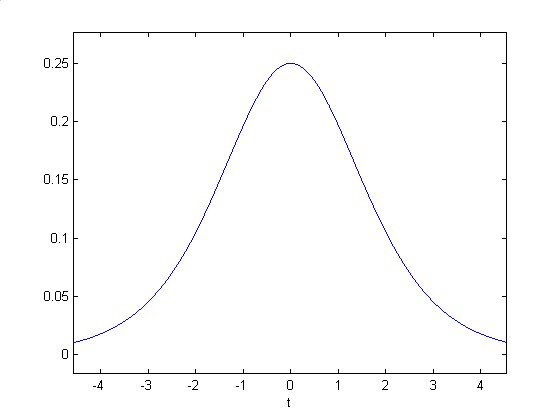Select Page

# The Bell Curve

The bell curve is derived from the pattern shown in Figure One. The curve in Figure One is well known because it is a good approximation of data that are obtained from several different sources. In the business world it is called the logistic curve, in mathematical circles it is called a sigmoid. This curve provides a good approximation of how people respond to incremental increases in sound pressure or the brightness of light.

One method of obtaining a sigmoid is to play a tone very softly at first and then to
increase the sound level in small increments while a listener indicates when there is a
perceived increase in loudness. The perception of loudness is well represented on the
vertical axis when the actual increase in sound pressure is presented along the
horizontal axis. Starting at the lower left of the graph each incremental change in
sound level makes very little difference in perceived loudness. As the sound pressure
increases, towards the middle of the graph, small changes make a larger difference in
perceived loudness. Accordingly, the curve is steeper. After a certain level of loudness is
obtained any additional increase makes very little difference in the perceived
loudness.

The pattern of data shown in Figure One is similar to the pattern that is obtained with
the visual system as the brightness of light is varied from very dim-on the left side of
the graph, to very bright-on the right side of the graph.Figure One. Response Curve. The sigmoid in this figure is defined by the equation,Where: A=B=k=1.

The bell-shaped curve shown in Figure Two is derived from the response curve shown in Figure One. This bell-shaped curve plots the rate of change in the slope of the idealized response curve of Figure One. The bell curve is the first derivative or f-prime of the response curve shown in Figure One.Figure Two. The normal distribution (f’), derived from the response curve shown in Figure One.